## Confidence Intervals and Margin of Error using the TiNSpire CX

To find Confidence Intervals of Proportions using Statistics Made Easy at  https://www.tinspireapps.com/?a=SPME use option 7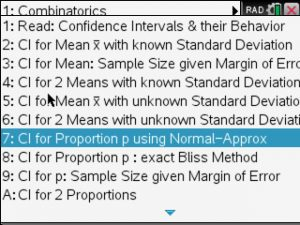Next, the given proportion po , sample size n and the confidence level alpha as shown below: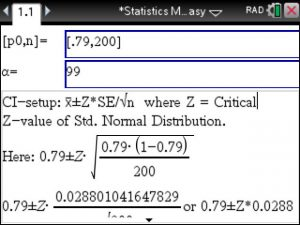Automatically, the correct setup and solution shows in the bottom window.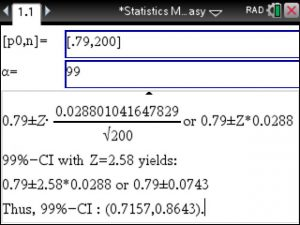To also find the sample size given the Margin of Error use option 9: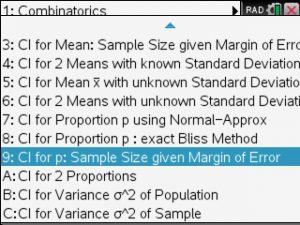you will enter the given Margin of Error, proportion and the confidence level Alpha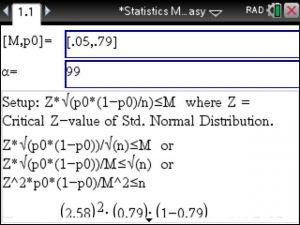Again, the correct setup is shown and the sample size is computed step by step, here we get n>=441.7187Posted on Categories statistics

## Confidence Interval for Proportions using the exact Bliss Method on the TiNspire

When finding Confidence Intervals for Proportions we typically involve the Normal Distribution to create an confidence Interval estimate.

However, this method requires the conditions np<5 and n(1-p)<5 to be fulfilled.

It is not uncommon to have a large sample size n available which likely disables this method.

This is where the Bliss Method comes in to find the exact Confidence Interval on the TiNspire.